# Mensuration Formulas for O-Level E Math

Dive into our list of Mensuration Formulas, to make sure you can solve Mensuration problems with ease in your O-Levels E-Math papers with this formula sheet.

Before you read on, you might want to download this entire formula sheet in PDF format to print it out, or to read it later.
This will be delivered to your email inbox.## Perimeter

1. We use millimetres (mm), centimetres (cm), metres (m) and kilometres (km) as units for measuring lengths or distances.

The units are related as follows:

1km = 1000m; 1m = 100cm; 1cm = 10mm

2. Perimeter of a circle is referred to as the circumference. The circumference, C, of a circle of radius, r, can be obtained by using this formula, circumference = 2 × π × r where π = 3.14 or 227.## Area

3. Area of a closed figure is the amount of space it covers. It is measured in square units.

4. Note:

a) 1 cm2 = 1 cm × 1 cm

= 10 mm × 10 mm

= 100 mm2

b) 1 m2 = 1 m × 1 m

= 100 cm × 100 cm

= 10 000 cm2

c) 1 km2 = 1 km × 1 km

= 1 000 m × 1 000 m

= 1 000 000 m2

Hectare (ha) is a unit for measuring large land areas such as farms.

1 ha = 10 000 m2

5. Area of a triangle = 12 × base × height6.  Area of a parallelogram =  base × height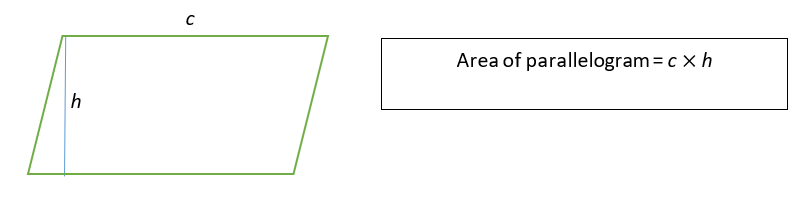7. Area of a trapezium = 12 × (sum of parallel sides) × height8. Area of a circle = π × r2, where π = 3.14 or 227.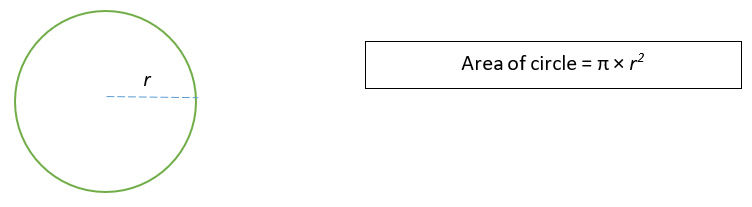## Volume

Volume of an object is the amount of space it occupies.  We use cubic metre (m3), cubic centimetre (cm3) and millimetre (mm3) as units for measuring volumes.

9. Note:

a) 1 cm3 = 1 cm × 1 cm × 1 cm

= 10 mm × 10 mm × 10 mm

= 1000 mm3

b) 1 m3 = 1 m × 1 m × 1 m

= 100 cm × 100 cm × 100 cm

= 1 000 000 cm3

c) 1 km3 = 1 km × 1 km × 1 km

= 1 000 m × 1 000 m × 1 000 m

= 1 000 000 000 m3

10. Volume of a cuboid = length × breadth × height11. Volume of a cube = length × length × length12. A cylinder is a special prism with a circular cross-section. Hence, its volume can be found by multiplying the area of the circular base by its height.

Volume of cylinder = Πr2h## Surface Area

13. A cube has 6 equal faces. Therefore, its surface area is obtained by multiplying the area of one face by 6.14.The lateral surface of a cylinder is also called the curved surface. If we rolled up a piece of rectangular sheet, the rectangular sheet will become the curved surface of the cylinder.Area of rectangle = curved surface area of the cylinder

= 2πrh

Hence, total surface area of a solid cylinder = area of curved surface + 2 × area of base

= 2πrh + 2πr2

## Volume and Surface Area of a Pyramid

A pyramid is a solid that has a base with a perpendicular vertex and slant lateral faces.

The base can be any polygon with 3 sides or more (triangle, a square, or a rectangle, etc.)Volume of pyramid = 13 × base area × height                          Base area =

Surface area of 4 sides = 12 × s × l × 4 sides

= 2s

Surface area of pyramid = 12 × base area + total area of slant ∆ faces

= s2 + 2sl

## Volume and Surface Area of a Cone

A cone is a solid with a circular base and a vertex.Arc length = circumference of circular base
Curved surface area of cone = πrl

Volume of cone = 13πr2

Surface area of a cone
= Area of circle + Area of curved surface

= πr2 + πrl

l = length of slant height =√(r2+h2)

## Volume and Surface Area of a Sphere and Hemisphere (Half-Sphere)

Every point on the surface of a sphere is equidistance from the centre.

A hemisphere is half a sphere.Volume of sphere = 43πr3

Surface area of a sphere = 4πr2

Volume of hemisphere = 12  × volume of sphere = 23πr3

Surface area = 12  × spherical surface area + area of circle

= 2πr2+ πr= 3πr2

## Volume and Surface Area of a Prism

Volume of prism = Base area × Height

Or  Area of cross-section × Length

Surface area of a prism = Area of all the faces

Or 2 × Cross-Sectional Area + (Perimeter of Cross Section) × length of prismVolume of triangular prism = 12 × b × h × l

Radian is another unit of measure for angles, similar to degrees.

One radian is the angle made at the center of a circle by an arc whose length is equal to the radius of the circle.#### Conversion from radians and degrees

π radians = 360°2 = 180°

#### Conversion between radians and degrees## Part of a Circle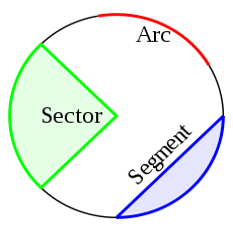## Arc Length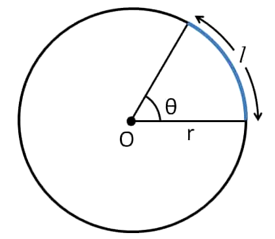If θ is measured in degree then arc length = θ°360° × 2πr

If θ is measured in radian then arc length = θ° × 2πr = rθ

## Area of SectorArea of circle = πr²

If θ is measured in degree then area of sector = θ°360 × πr² = rθ

If θ is measured in radian then area of sector = θ° × πr² = 12 r²θ

## Area of Segment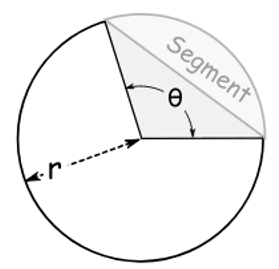Area of segment = Area of Sector ‒ Area of Triangle

= θ°360° × πr² ‒ 12 r² sinθ  (if θ is a degree)

= 12 r²θ ‒12 r²sinθ  (if θ is a radian)

Important: If angles are in radian, change the calculator to radian mode.

For more mensuration formulas and tips, check out our free math eBooks and test papers for secondary school students!

## Check out our exam guide on other topics!

Before you go, you might want to download this entire formula sheet in PDF format to print it out, or to read it later.

This will be delivered to your email inbox.Mr Tan Kok Heng
NIE Trained
Math Specialist
Jimmy Maths and Grade Solution Learning Centre

## Does your child need help in his or her studies?

### 1) Live Zoom Lessons at Grade Solution Learning CentreAt Grade Solution Learning Centre, we are a team of dedicated educators whose mission is to guide your child to academic success. Here are the services we provide:

– Live Zoom lessons
– EdaptIQ™, a smart learning platform that tracks your child’s progress, strengths and weaknesses through personalised digital worksheets.

We provide all these services above at a very affordable monthly fee to allow as many students as possible to access such learning opportunities.

We specialise in English, Math, and Science subjects.

You can see our fees and schedules here >>

Primary Math Tuition

Secondary Math Tuition

### 2) Pre-recorded Online courses on Jimmymaths.comIf you are looking for something that fits your budget, or prefer your child learn at his or her own pace, you can join our pre-recorded online Math courses.